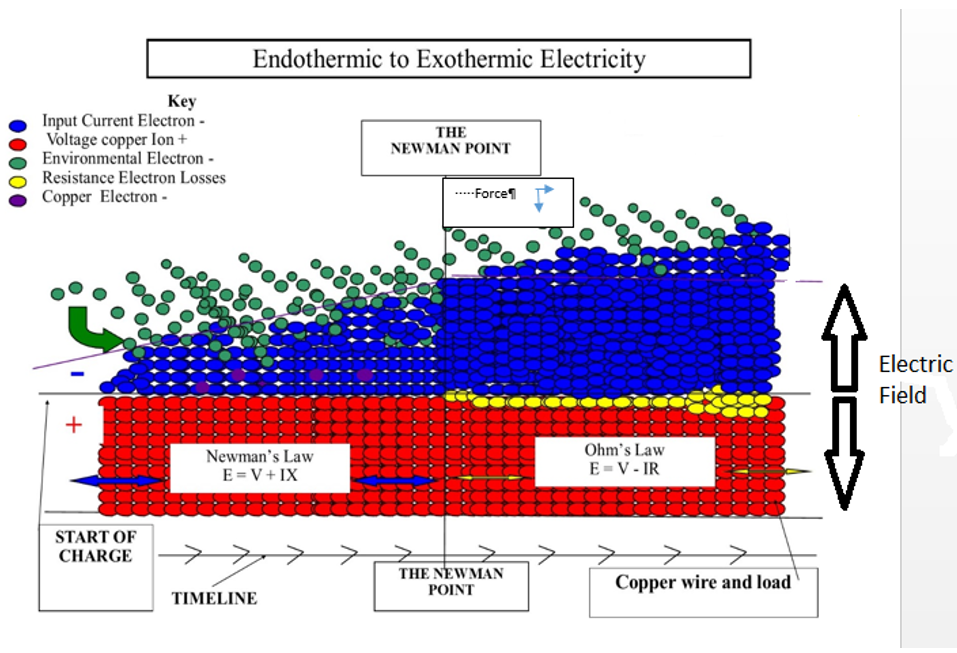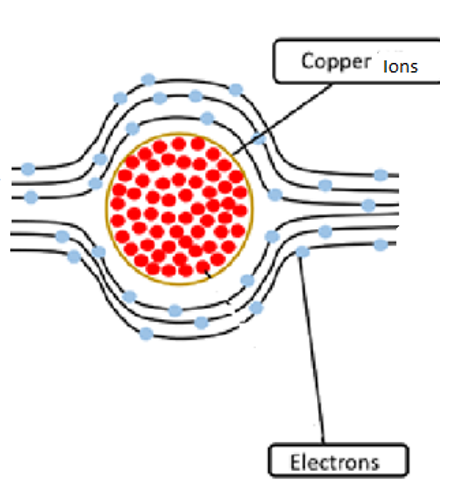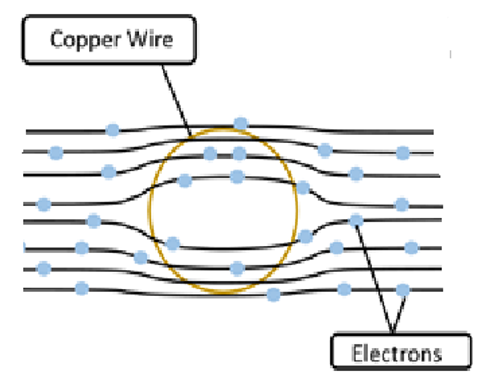Endothermic to Exothermic reaction in a copper wire

EMF output = V x IX             Endothermic (Equation 10)

EMF output = V – IR              Exothermic ( Equation 11)

X = parameter heat gain constant for a specific design of a system.

## The Newman Point

At the start of an electric field charge, a force in is applied. This force applied can be administered to a system in a variety of ways, for example, wind power, solar, steam pressure, electrical force, etc. The EMF then becomes a force out of the atom ions due to the Photoelectric Effect taking place, and repulsion and attraction forces now dominating the state of the system. The force in is the current traveling to the load and the wire, the negative part of the electric field will continue to grow with order and equidistant spacing of charge carriers within the field due to like repulsion, and will eventually equal the joules force out of the atom ions. This, I have named, ‘The Newman Point’, the point in time of an electric field charge when the negative current field, force in, equals the atom ions force out. This is the point in time during the charge before any frictional forces are introduced, the system is balanced, and the electric field is charged above 100% efficiency. The circuit of electric field charge is completed within the system.

After the Newman point, if more current is placed into the system resistance is met. This is by an invasion of force in (current) into the atom ions force out. This leads to the next observation:

### Newman’s law

There will be no resistance in an electric field charge, until the electric field current has a greater force in than the voltage atom ions field force out of the system.Represents the endothermic equation, the electric field current growth stage, ‘force in’ is less than ‘force out.’Represents the exothermic equation, the force in is greater than the force out, this is the electric field current invading the voltage atom ions causing frictional force, this occurs after the Newman Point.